# 10 Most Powerful Militaries in The World

1
2222Related eBooks

Let us take a look at the 10 most powerful militaries of the world. The list has been compiled after thorough research of the defense forces of approximately 68 countries in terms of manpower, air power, geography, finances, resources, account manpower and many other important factors.

So, let’s take a look at the complied look. We know that military of a nation is the most curtail thing for it. Every country tries to give the maximum resources to them so that the military of the nation is the strongest.

1. Brazil.The budget allotted for defense is \$31,576,000,000. The total active military personnel is 371,199 with labor force of 104,700,000. The total aircraft strength is 822 and of navy is 106.

After, weighing all the parameters, the power index comes out to be 0.6912.

1. Italy.The budget allotted for defense is \$31,946,000,000. The total active military personnel is 293,202 with labor force of 25,080,000. The total aircraft strength is 770 and of navy is 179.

After, weighing all the parameters, the power index comes out to be 0.6838.

1. South Korea.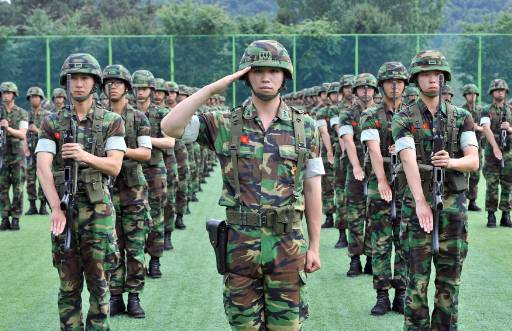The budget allotted for defense is \$28,280,000,000. The total active military personnel is 653,000 with labor force of 25,100,000. The total aircraft strength is 925 and of navy is 190.

After, weighing all the parameters, the power index comes out to be 0.6547.

1. Germany.The budget allotted for defense is \$43,478,000,000. The total active military personnel is 148,996with labor force of 43,620,000. The total aircraft strength is 871 and of navy is 67.

After, weighing all the parameters, the power index comes out to be 0.6491.

1. France.The budget allotted for defense is \$58,244,000,000. The total active military personnel is 362,485 with labor force of 29,610,000. The total aircraft strength is 544 and of navy is 180.

After, weighing all the parameters, the power index comes out to be 0.6163.

1. The United Kingdom.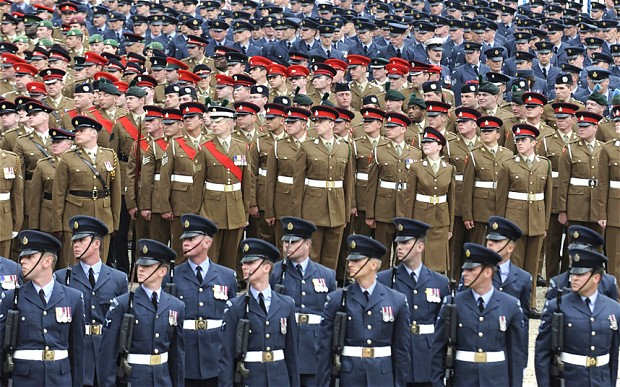The budget allotted for defense is \$57,875,170,000. The total active military personnel is 224,500 with labor force of 31,720,000. The total aircraft strength is 1,412 and of navy is 77.

After, weighing all the parameters, the power index comes out to be 0.5185.

1. India.The budget allotted for defense is \$44,282,000,000. The total active military personnel is 1,325,000 with labor force of 487,600,000. The total aircraft strength is 1,962 and of navy is 170.

After, weighing all the parameters, the power index comes out to be 0.4346.

1. China.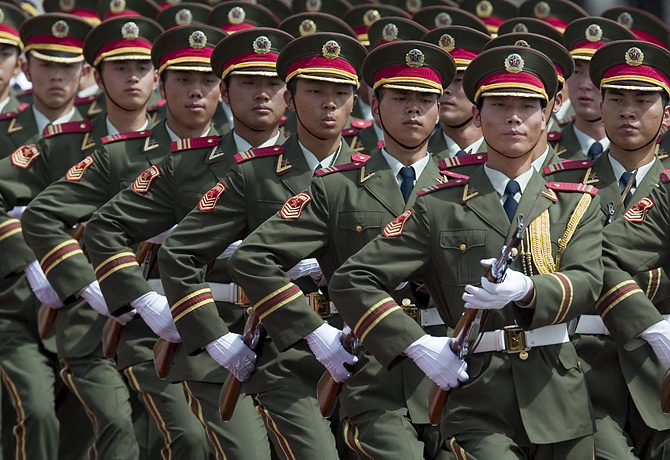The budget allotted for defense is \$129,272,000,000. The total active military personnel is 2,285,000 with labor force of 795,500,000. The total aircraft strength is 5,048 and of navy is 972.

After, weighing all the parameters, the power index comes out to be 0.3351.

1. Russia.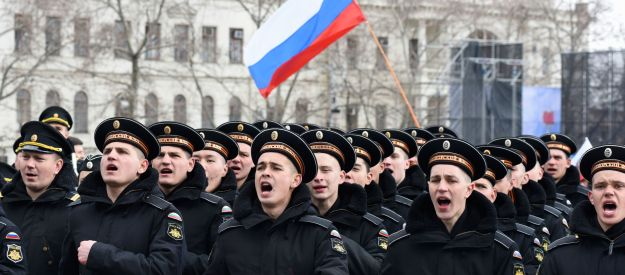The budget allotted for defense is \$64,000,000,000. The total active military personnel is 1,200,000 with labor force of 75,330,000. The total aircraft strength is 4,498 and of navy is 224.

After, weighing all the parameters, the power index comes out to be 0.2618.

1. United States.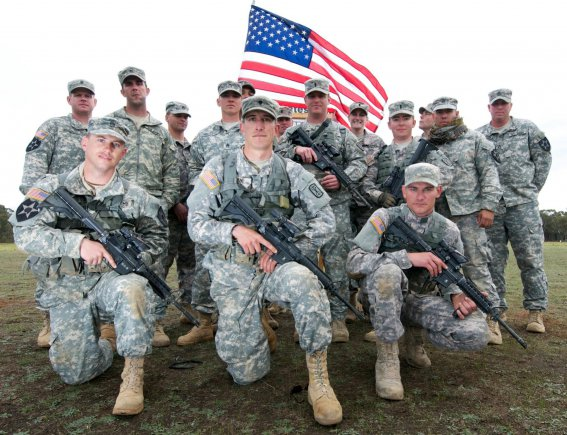The budget allotted for defense is \$689,591,000,000. The total active military personnel is 1,477,896 with labor force of 153,600,000. The total aircraft strength is 15,293 and of navy is 290.

After, weighing all the parameters, the power index comes out to be 0.2475.

Conclusion

So where does your country stand? Are you proud of your army? If yes type ‘yes with your country name’ in the comment section and show how proud you are of your country. Nuclear capabilities are not included in this calculation.